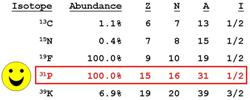# Delta G

This parody (written by Greg Crowther) is sung to the tune of "Delta Dawn" (written by Alex Harvey and Larry Collins and performed by Helen Reddy).

 Context

This song provides a concise introduction to Gibbs free energy (ΔG) and the equation by which it is calculated (ΔG = ΔH - TΔS).

 Lyrics

CHORUS:
Delta G -- plus or minus it will be
For reactions in conditions that are known.
If delta H is less than T times delta S,
The reaction can go forward on its own.

The Gibbs free energy gives me satisfaction
From gauging the spontaneity of reactions.
Its equation has an "H" -- that's enthalpy --
And entropy is "S" and temperature's "T."

The equation shows if reactions can proceed,
But it doesn't say a thing about their speed.
The study of those rates is called Kinetics,
And it uses lots more math that'll give you headaches.

CHORUS